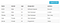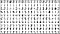# Building Machine Learning Models on the Web with TensorflowJS — Part 1

TensorFlow is a highly-known open-source software library meant to process dataflow and make predictions based on machine learning models. However, for the longest time, TensorFlow has been restricted as a “python-oriented” software, limiting the use of the library for websites. This has caused many developers to resort to creating machine learning algorithms from scratch for web-pages, until their voices have been heard and thus TensorFlowJS was born.

Today I’ll be covering Part 1 of a brand new series dedicated to the ins and outs of TensorFlowJS, and creating machine learning models in the web environment. In this blog we will be covering the basics of machine learning and give a brief introduction to TensorflowJS itself, as well as resources that can be used to further understand the library. Let’s get started.

# What is TensorflowJS?

Before we dive too deep, let’s first understand what TensorflowJS actually is:

TensorflowJS, like Tensorflow, is a library that is able to build, train, and makes predictions from machine learning models, but in a Javascript environment. TensorflowJS has the ability to bring Python-built ML algorithms to function in a web-app by using Tensorflow Sequential, which acts similar to Keras, but built for Node.

# Understanding TensorflowJS:

## Datasets:

When it comes to training a machine learning model, one of the first steps is to find a set of examples to guide the model on what the expectation may be. These examples are normally contained in what is known as CSV files, which you could think of as a built-in table containing columns and rows of sample data, which is called a dataset.A visual of how a CSV dataset looks

Some datasets like XGBoost (which is used to predict malicious websites) require a CSV format since most data is compared based on statistics, while other datasets such as Mnist (dataset used to predict hand written integers) are stored in its most compressed form as a png file.A visual depiction of what the Mnist dataset looks like.

## Convolutionals:

Let’s say that the sample data we are going to use for our machine learning model is going to require the Mnist dataset, what we will need for our model to do next is read our sample data, but how can our model see the image? Everything a computer can see is made up of integers, so if the model were to break down each pixel of the image into integers, then the model will be able to read the image based on the values of each pixel. This process is known as a convolutional, where pixel units of an image are the sum of n by n slots.

Let’s break down this visual depicting a convolutional taking place:

Let’s narrow down and focus on the process of the first selected units of 9. Notice how in the center there is a 9 unit square known as a “kernel”. If we were to do the math for the first 9 slots in the first square, the kernel will multiply the values of all units and then sum up the results. So automatically 121 will be negated since you get nothing when multiplying it by zero while 73 and 113 will be -186 because the kernel multiplies them by -1, and finally 60 will be multiplied by 5 to get 300, and if we were to get the sum, we will have 114, which is the result value placed in the last square. That’s how convolutionals work!

## Neural Networks:

Now that we know what datasets are and how the model will read the sample data using convolutions, let’s take a look at the training phase a machine learning model will take. If we were to analyze the visual depiction below, we will notice how all pixel instances read will be plugged into one of the circles to the very right. This is what is known as the “hidden layer”, which is where the convolutionals take place.

The structure of a neural network can also often vary. There are numerous varieties based on either what datasets the model is training for, how advanced the model is going to be, if the model will be comparing from a built in database, or maybe it’s just the developer’s preference.

## Losses:

For the machine learning model to understand the do-s and don’t-s, the model must first compare input values and determine if the prediction is good or bad based on a decimal grade. Depending on how far or close the value is to the whole number 1 will determine if the prediction is either good or bad. If the grade is considered bad, then the model will be penalized and scrap the instance entirely, but if the grade is good, then the grade will be recorded for future training instances. This is how the model learns and adapts per epoch (number of training instances set).

## Optimizers:

Once each instance in the hidden layer resolves its convolutionals, the model moves onto the next phase in training; learning between what is considered right and what is wrong, and balances the weight and learning rate of your model. This method is known as an optimizer, which is used to find the cross entropy (the comparison between two probable outcomes and gathers loss sum) and the generalization (the process of creating correct predictions for new data) based on the type of optimizer used (such as adam or adagrad), and the conditions of previous losses to prevent future losses from occurring.

## Gradient Descent:

Lastly, after the Optimizer finishes its course, the model’s final stage of training phase is to map the results from the optimized instances and finds the local minima (lowest projected value) in the graph of instances. This is known as a Gradient Descent, which is used to automatically tweak the weights and biases of the model. This is how the model checks for accuracy between all instances and reaches the most viable result.

# Conclusion:

Within the course of this blog we have covered exactly what TensorFlowJS is, what a dataset is and how to read visual data using convolutionals, what neural networks are and how they compare results and losses, what optimizers are and how they can gather the sum of losses to prevent future losses, and what gradient descent is and how it’s used to map the results of training and testing data from a model. Hopefully you learned more about machine learning and TensorFlowJS when leaving this blog than entering, and be sure to follow the next part whenever it is released to see how we can use what we taught here in this blog to create an app using TensorFlowJS.

## Resources:

Kaggle: For open-source datasets.

JS Dev